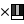# RGBO Multiply node

Left Toolbar >Math Nodes >Rgbo Multiply

The RGBO Multiply node allows to multiply the components of the Rgbo value by a number. The checkboxes allow you to select to which components the multiplication is applied to.

## Procedure

### To generate a Rgbo Multiply node in the node graph:

1. On the left toolbar choose Point Nodes> Rgbo Multiply2. Drag the Rgbo Multiply icon into the Node Graph.3. Connect the Output .

## Inputs

rgbo_a A RGBO type.

rgbo_b A RGBO type.

## Outputs

result A RGBO type.

## Attributes PanelThese attributes are exposed as input by default. Right-click the Node to hide the attribute as input and set the point values manually. Default R:0.0, G:0.0, B:0.0, O:1.0

rgbo A

rgbo B

Math Nodes

Main Page